Skip to main content

# Robust design of Farrow-structure-based steerable broadband beamformers with sparse tap weights via convex optimization

## Abstract

The Farrow-structure-based steerable broadband beamformer (FSBB) is particularly useful in the applications where sound source of interest may move around a wide angular range. However, in contrast with conventional filter-and-sum beamformer, the passband steerability of FSBB is achieved at the cost of high complexity in structure, i.e., highly increased number of tap weights. Moreover, it has been shown that the FSBB is sensitive to microphone mismatches, and robust FSBB design is of interest to practical applications. To deal with the aforementioned problems, this paper studies the robust design of the FSBB with sparse tap weights via convex optimization by considering some a priori knowledge of microphone mismatches. It is shown that although the worst-case performance (WCP) optimization has been successfully applied to the design of robust filter-and-sum beamformers with bounded microphone mismatches, it may become unapplicable to robust FSBB design due to its over-conservativeness nature. When limited knowledge of mean and variance of microphone mismatches is available, a robust FSBB design approach based on the worst-case mean performance optimization with the passband response variance (PRV) constraint is devised. Unlike the WCP optimization design, this approach performs well with the capability of passband stability control of array response. Finally, the robust FSBB design with sparse tap weights has been studied. It is shown that there is redundancy in the tap weights of FSBB, i.e., robust FSBB design with sparse tap weights is viable, and thus leads to low-complexity FSBB.

## Introduction

As one of the key technologies for microphone arrays, broadband beamforming has been used in a wide range of audio and speech processing applications, such as teleconferencing, hearing aids, and audio surveillance . The most popular methods for broadband beamforming for microphone arrays are based on the well-known filter-and-sum structure . In practice, sound source of interest may move around some angular range. Accordingly, the passband width of a broadband beamformer usually needs to be designed to cover the whole angular range of movement of the sound source.1 It is known that, there is a trade-off between passband width and stopband attenuation for a filter-and-sum broadband beamformer, i.e., the larger the passband width, the worse the stopband attenuation, and vice versa.2 As a result, the spatial filtering performance of filter-and-sum broadband beamformers will deteriorate greatly when the sound source is moving around a wide angular range. To combat this problem, one promising solution is to design steerable broadband beamformers, where their passbands can be adjusted dynamically with a simple scheme, with no need of redesign of the broadband beamformers.

Recent years have seen great interest in the design of steerable broadband beamformers for microphone arrays . Among the proposed design approaches, some are tailored to specific array configurations, such as differential microphone arrays  and spherical microphone arrays . Comparatively, the Farrow-structure-based steerable broadband beamformers (FSBBs), also known as the polynomial beamformers , are particularly interesting in some applications, since they are applicable to arbitrary array configurations, and moreover, their passbands can be steered online with just one single parameter . In practice, there usually exist some mismatches among microphones, such as gain and phase errors . Unfortunately, the FSBBs are highly sensitive to microphone mismatches. Therefore, the design of FSBBs robust against microphone mismatches has drawn attention recently.

Generally speaking, according to whether any priori knowledge of microphone characteristics is used or not, the existing design approaches for robust FSBBs can be classified into two categories. In [9, 12], white noise gain (WNG) constraint has been utilized to design robust FSBBs, where no knowledge of microphone characteristics is considered. However, the main problem with the WNG constraint-based approach is that it is unclear how to choose the WNG constraint level optimally. To get over the problem, a robust FSBB design approach based on the weighted least squares has been proposed in , which takes into account the probability density function (PDF) of microphone characteristics. By considering the knowledge of microphone characteristics, no user-tuning parameters are required any more; thus, it can facilitate the FSBB design. Although this design approach has shown robust against microphone mismatches, the difficulty with the approach is that the PDF of microphone characteristics may not be easily accessible in practice. Instead, the bounds of uncertain microphone mismatches [16, 17] or the limited knowledge of mean and variance of microphone mismatches  may be practically available to a designer. Therefore, it is necessary to establish efficient design schemes for robust FSBBs by considering these types of knowledge of microphone characteristics. Besides the aforementioned robustness problem of the FSBB in the presence of microphone mismatches, another problem with the FSBB is that its computational complexity is particularly demanding in contrast with its counterpart based on filter-and-sum structure, which is the price it has paid for the passband steerability. However, the low-complexity FSBB design has not been addressed in the literature, which is of interest to practical applications.

Inspired by our previous work on robust filter-and-sum beamformer design [16, 18], the robust FSBB design using convex optimization with some priori knowledge of microphone mismatches is studied in this paper. Moreover, to reduce the computational complexity of the robust FSBB, the robust FSBB design with sparse tap weights has also been studied. To summarize, the contributions of the paper are threefold:

• For bounded microphone mismatches, the robust FSBB design based on the worst-case performance (WCP) optimization criterion has been established. It is shown that although the WCP optimization has been successfully applied to the design of robust filter-and-sum beamformers as in [16, 17]; unfortunately, it may become unapplicable to robust FSBB design due to its over-conservativeness nature as analyzed in the paper.

• When limited knowledge of mean and variance of microphone mismatches is available to a designer, the robust FSBB design approach based on the worst-case mean performance (WCMP) optimization with the passband response variance (PRV) constraint is developed. Unlike the WCP optimization-based design, the proposed approach performs well for robust FSBB design with the capability of passband stability control of array response. Moreover, some insights into the properties of the PRV of robust FSBB have also been revealed.

• In contrast with filter-and-sum beamformer, the passband steerability of FSBB is achieved at the cost of high complexity in structure, i.e., highly increased number of tap weights. However, it is shown that there is redundancy in tap weights of FSBB, i.e., robust FSBB design with sparse tap weights is viable. To this end, a two-stage approach for the design of robust FSBB with sparse tap weights using the reweighted l 1-norm constraint optimization has been proposed, which leads to the design of low-complexity FSBB.

The rest of the paper is organized as follows. In Section 2, we formulate the problem of robust FSBB design. In Section 3, we present the robust FSBB design using the WCP optimization, when the bounds of microphone mismatches are known. In Section 4, we develop the robust FSBB design using the WCMP optimization with the PRV constraint, when the limited knowledge of mean and variance of microphone mismatches is available. In Section 5, the robust FSBB design with sparse tap weights is studied. Design examples are presented in Section 6 to illustrate the performance of the proposed approaches. Finally, Section 7 concludes the paper.

## Problem formulation

Consider a K-element linear microphone array in the farfield, where the distance the kth microphone and the center of the array is denoted by d k . The configuration of the FSBB is shown in Fig. 1. Unlike the well-known filter-and-sum beamformers, herein, a Farrow structure consisting of M finite-impulse-response (FIR) subfilters is used behind each microphone, where the tap length of each FIR subfilter is N. The beampattern of the FSBB at frequency f and angle of arrival θ (defined with respective to the array axis, θ(0,180°)) can be expressed as 

{} {\fontsize{7.8pt}{9.6pt}\selectfont{\begin{aligned} P(\phi_{d},f,\theta)=\!\sum\limits_{k=0}^{K-1}\sum\limits_{m=0}^{M-1}\sum\limits_{n=0}^{N-1}A_{k}\left(\,f,\theta\right)w_{k,n,m}e^{-j2\pi fd_{k}\cos\theta/c}e^{-j2\pi fn/f_{s}}D^{M-1-m} \end{aligned}}}
((1))

where $$A_{k}\left (\,f,\theta \right)=\left [1+a_{k}\left (\,f,\theta \right)\right ]e^{-j\gamma _{k}\left (\,f,\theta \right)}$$ with a k (f,θ) and γ k (f,θ) being the gain and phase errors of the kth microphone, w k,n,m denote the weights of the FSBB, c is the speed of sound, f s represents the sampling frequency, and D=(ϕ d −90°)/90° with ϕ d being the desired steering direction of the FSBB.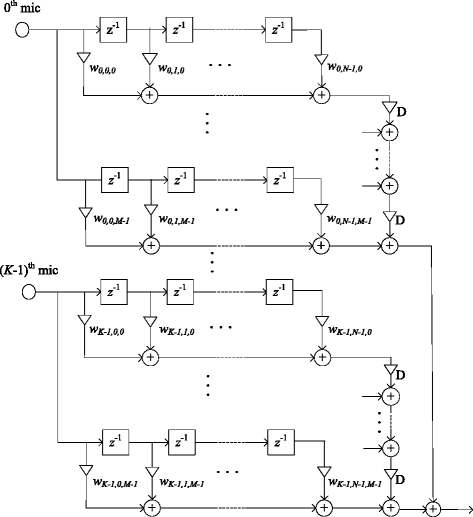Fig. 1

To simplify notation, (1) can be rewritten in the vector form

$$P(\phi_{d},f,\theta)=\mathbf{w}^{T}\mathbf{\overline{g}}(\phi_{d},f,\theta)$$
((2))

where (·)T denotes the transpose, w=[w 0,0,0,,w 0,0,M−1,w 0,N−1,0,,w 0,N−1,M−1,,w K−1,N−1,0,,w K−1,N−1,M−1]T is the weight vector of the FSBB, and $$\mathbf {\overline {g}}(\phi _{d},f,\theta)$$ is the array steering vector, which is given by

$$\mathbf{\overline{g}}\left(\phi_{d},f,\theta\right)=\left(\mathbf{A}\left(\,f,\theta\right)\odot\mathbf{d}\left(\,f,\theta\right)\right)\otimes \mathbf{e}\left(\,f\right)\otimes \mathbf{s}(D)$$
((3))

with

$$\mathbf{A}\left(\,f,\theta\right)=\left[A_{0}\left(\,f,\theta\right),\cdots,A_{K-1}\left(\,f,\theta\right)\right]^{T}$$
((4))
$$\mathbf{d}\left(\,f,\theta\right)=\left[e^{-j2\pi fd_{0}\cos\theta/c},\cdots,e^{-j2\pi fd_{K-1}\cos\theta/c}\right]^{T}$$
((5))
$$\mathbf{e}\left(\,f,\theta\right)=\left[1,e^{-j2\pi f/f_{s}},\cdots,e^{-j2\pi f(N-1)/f_{s}}\right]^{T}$$
((6))
$$\mathbf{s}(D)=\left[D^{M-1},\cdots,D,1\right]^{T}$$
((7))

where denotes the Hadamard product, and denotes the Kronecker product.

Given some priori knowledge on microphone characteristics A(f,θ) and a desired response P d (ϕ d ,f,θ), our problem is to design an optimal robust beamformer weight vector w using some criterion such that the beamformer response P(ϕ d ,f,θ) can optimally fit P d (ϕ d ,f,θ) over the predefined frequency-angle range of interest (f,θ)Ω and the predefined steering direction range of interest ϕ d Ψ(0,180°). The advantage of the FSBB is that its passband can be steered towards arbitrary directions with no need of redesign of beamformer weight vector.

## Robust FSBB design using the WCP optimization

In this section, we study the robust FSBB design via convex optimization by using the WCP optimization in the case of bounded microphone mismatches. To proceed, first, we need to introduce a non-robust design approach using minimax criterion when there are no microphone mismatches.

### Non-robust design

When there are no microphone mismatches, the microphone characteristics now become A k (f,θ)=1,(k=0,,K−1). Accordingly, (3) reduces to

$$\mathbf{\overline{g}}\left(\phi_{d},f,\theta\right)=\mathbf{d}\left(\,f,\theta\right)\otimes \mathbf{e}\left(\,f\right)\otimes \mathbf{s}(D)\triangleq \mathbf{g}\left(\phi_{d},f,\theta\right)$$
((8))

where g(ϕ d ,f,θ) denotes the steering vector without microphone mismatches.

The problem for FSBB design using the minimax criterion can be formulated as

$$\min\limits_{\mathbf{w}} \max\limits_{(f,\theta)\in \mathbf{\Omega}}\max\limits_{\phi_{d}\in \mathbf{\Psi}}\left|\mathbf{w}^{T}\mathbf{g}(\phi_{d},f,\theta)-P_{d}(\phi_{d},f,\theta)\right|,$$
((9))

which can be recast as the following semi-indefinite convex programming

$$\min\limits_{\epsilon,\mathbf{w}} ~~\epsilon ~~~\mathrm{s.t.}\left\{ \begin{array}{l} \left|\mathbf{w}^{T}\mathbf{g}\left(\phi_{d},f,\theta\right)-P_{d}\left(\phi_{d},f,\theta\right)\right|\leq\epsilon \\ \left(\,f,\theta\right)\in \mathbf{\Omega}, \phi_{d}\in \mathbf{\Psi}. \end{array} \right.$$
((10))

The above problem can be further formulated as a second-order cone programming (SOCP) problem and thus can be solved efficiently via the interior point methods [19, 20].

### Robust design

Now, we consider the robust design of FSBB in the presence of bounded microphone mismatches by using the WCP optimization-based criterion. Due to microphone mismatches, there will exist some perturbation in the steering vector of FSBB, i.e., $$\Delta \mathbf {g}(\phi _{d},f,\theta)=\mathbf {\overline {g}}(\phi _{d},f,\theta)-\mathbf {g}(\phi _{d},f,\theta)$$. Assume |a k (f,θ)|≤δ a <1 and |γ k (f,θ)|≤δ γ <π/2, where δ a and δ γ are the known bounds. Regarding the perturbation of the steering vector of FSBB, we have the following proposition.

### Proposition1.

The perturbation of the steering vector of FSBB is bounded by

{} {\fontsize{9.2pt}{9.6pt}\selectfont{\begin{aligned} &\|\Delta\mathbf{g}(\phi_{d},f,\theta)\|\leq \\&\sqrt{KN\cdot\underset{\phi_{d}\in \mathbf{\Psi}}{\max}\left\{\frac{1-D^{2M}}{1-D^{2}}\right\}\cdot\left\{(1+\delta_{a})^{2}-2(1+\delta_{a})\cos{\delta_{\gamma}}+1\right\}}. \end{aligned}}}
((11))

### Proof.

Using (3) and (8), and noting that |D|<1, it holds that

$$\begin{array}{@{}rcl@{}} &&\left\|\Delta\mathbf{g}(\phi_{d},f,\theta)\right\|\\ &=&\left\|\left[\mathbf{A}\left(\,f,\theta\right)\odot\mathbf{d}\left(\,f,\theta\right)-\mathbf{d}\left(\,f,\theta\right)\right]\otimes \mathbf{e}\left(\,f\right)\otimes \mathbf{s}(D)\right\| \end{array}$$
{} {\fontsize{8.8pt}{9.6pt}\selectfont{\begin{aligned} &=\sqrt{N\cdot\frac{1-D^{2M}}{1-D^{2}}\cdot\sum\limits_{k=0}^{K-1}(A_{k}\left(\,f,\theta\right)-1)^{2}}\\ &=\sqrt{N\cdot\frac{1-D^{2M}}{1-D^{2}}\cdot\sum\limits_{k=0}^{K-1}\left\{(1+a_{k})^{2}-2(1+a_{k})\cos{\gamma_{k}}+1\right\}} \\ &\leq\sqrt{KN\cdot\underset{\phi_{d}\in \mathbf{\Psi}}{\max}\left\{\frac{1-D^{2M}}{1-D^{2}}\right\}\cdot\left\{(1+\delta_{a})^{2}-2(1+\delta_{a})\cos{\delta_{\gamma}}+1\right\}}. \end{aligned}}}

The design of robust FSBB with the WCP optimization can be formulated as

{} {\fontsize{8.4pt}{9.6pt}\selectfont{\begin{aligned} \min\limits_{\mathbf{w}} \max\limits_{(f,\theta)\in \mathbf{\Omega}}\max\limits_{\phi_{d}\in \mathbf{\Psi}}\max\limits_{\Delta\mathbf{g}}\left|\mathbf{w}^{T}\left[\mathbf{g}(\phi_{d},f,\theta)+\Delta\mathbf{g}(\phi_{d},f,\theta)\right]-P_{d}(\phi_{d},f,\theta)\right|\!. \end{aligned}}}
((12))

With Proposition 1, the problem (12) can be reformulated as the following minimax problem

$$\min\limits_{\mathbf{w}}\max\limits_{(f,\theta)\in \mathbf{\Omega}}\max\limits_{\phi_{d}\in \mathbf{\Psi}}\left|\mathbf{w}^{T}\mathbf{g}(\phi_{d},f,\theta)-P_{d}(\phi_{d},f,\theta)\right|+\varepsilon \|\mathbf{w}\|$$
((13))

where ε is chosen as the lower bound of Δ g given by (11). By introducing some auxiliary variables, (13) can be recast as the following convex optimization problem

$$\min\limits_{\epsilon,\varsigma,\mathbf{w}} ~~\epsilon ~~~\mathrm{s.t.}\left\{ \begin{array}{l} \left|\mathbf{w}^{T}\mathbf{g}(\phi_{d},f,\theta)-P_{d}(\phi_{d},f,\theta)\right| \leq\varsigma\\ \varepsilon \|\mathbf{w}\|\leq\epsilon-\varsigma\\ \left(\,f,\theta\right)\in \mathbf{\Omega}, \phi_{d}\in \mathbf{\Psi} \end{array} \right.$$
((14))

The procedures of the robust FSBB design using the WCP optimization are summarized in the following.### Remark1.

As we know, although the WCP optimization approach has been successfully used in the design of robust broadband beamformers with the filter-and-sum structure, it is conservative because the worst scenario that all microphone mismatch errors simultaneously attain their maximal values rarely occurs in practice. In contrast, the robust design of FSBB using the WCP optimization is more conservative since it just considers the more rarely occurred worst case, which requires not only that all microphone mismatch errors simultaneously attain their maximal values but also that the steering direction of the FSBB is at the boundary of the steering direction range of interest (note that (1−D 2M)/(1−D 2) in (11) achieves its maximal value when the steering direction is at the boundary of Ψ). As a result, the WCP optimization-based design for robust FSBB suffers from outstanding overconstraint problem which may lead to poor design performance.

## Robust FSBB design using the WCMP optimization with the PRV constraint

In this section, we study the robust FSBB design via convex optimization when the knowledge we have on microphone mismatches is only their bounded mean and variance.

### Robust design using the WCMP optimization

Suppose the mean values of microphone gain and phase mismatches are imprecisely known and are bounded by some known small constants μ a and μ γ respectively, i.e., $$|\mathbb {E}\{a_{k}(f,\theta)\}|\leq \mu _{a}$$, $$|\mathbb {E}\{\gamma _{k}(f,\theta)\}|\leq \mu _{\gamma }$$, where $$\mathbb {E}\{\cdot \}$$ denotes the mean value. Following the similar derivation as Proposition 1, it holds that the mean perturbation of the steering vector of the FSBB is bounded by

{} {\fontsize{9.2pt}{9.6pt}\selectfont{\begin{aligned} &\|\mathbb{E}\left\{\Delta\mathbf{g}(\phi_{d},f,\theta)\right\}\|\leq\\& \sqrt{KN\cdot\underset{\phi_{d}\in \mathbf{\Psi}}{\max}\left\{\frac{1-D^{2M}}{1-D^{2}}\right\}\!\cdot\!\left\{(1+\mu_{a})(1+\mu_{a}-2\cos\mu_{\gamma})+1\right\}}. \end{aligned}}}
((15))

The robust design for the FSBB using the WCMP optimization can be cast as

{} {\fontsize{8.2pt}{9.6pt}\selectfont{\begin{aligned} \underset{\mathbf{w}}{\min}\underset{(f,\theta)\in\mathbf{\Omega}}{\max}\underset{\phi_{d}\in \mathbf{\Psi}}{\max} \underset{\Delta\mathbf{g}}{\max} \left|\mathbb{E} \left\{\mathbf{w}^{T}\left[\mathbf{g}(\phi_{d},f,\theta)+\Delta\mathbf{g}(\phi_{d},f,\theta)\!\right]\!\right\}-P_{d}(\phi_{d},f,\theta)\right| \end{aligned}}}
((16))

Using (15), the WCMP optimization problem can be reformulated as

$$\min\limits_{\mathbf{w}}\underset{(f,\theta)\in\mathbf{\Omega}}{\max} \underset{\phi_{d}\in \mathbf{\Psi}}{\max} \left|\mathbf{w}^{T}\mathbf{\overline{g}}(\phi_{d},f,\theta)-P_{d}(\phi_{d},f,\theta)\right|+\overline{\varepsilon} \|\mathbf{w}\|$$
((17))

where $$\overline {\varepsilon }$$ is chosen as the lower bound of $$\|\mathbb {E}\{\Delta \mathbf {g}(\phi _{d},f,\theta)\}\|$$ given by (15). Alternatively, the optimization problem (17) can further be recast as the following SOCP problem

$$\min\limits_{\epsilon,\varsigma,\mathbf{w}} ~~\epsilon ~~~\mathrm{s.t.}\left\{ \begin{array}{l} \left|\mathbf{w}^{T}\mathbf{\overline{g}}(\phi_{d},f,\theta)-P_{d}(\phi_{d},f,\theta)\right| \leq\varsigma\\ \overline{\varepsilon} \|\mathbf{w}\|\leq\epsilon-\varsigma\\ \left(\,f,\theta\right)\in \mathbf{\Omega}, \phi_{d}\in \mathbf{\Psi} \end{array} \right.$$
((18))

### Remark2.

Like the WCP optimization-based design, the WCMP optimization-based design also belongs to the class of white noise gain constraint-based approaches. Consider the fact that μ a <δ a and μ γ <δ γ , it follows from (15) and (11) that $$\overline {\varepsilon }<\varepsilon$$. Therefore, the WCMP optimization-based design is less conservative than the WCP optimization-based design and hence is suitable for robust FSBB design as demonstrated by the simulation results in Section 6.

### Robust design incorporating the PRV constraint

To enhance the robustness of the FSBB, i.e., to improve its stability of passband response and hence to reduce target signal distortion, we hereby consider to incorporate the PRV constraint into the design procedures by using the bounded variances of microphone mismatches. To proceed, we make the following assumptions : 1) microphone gain and phase errors are uncorrelated; 2) all microphones have the same variances Var{a(f,θ)} and Var{γ(f,θ)} for gain and phase errors, respectively. The only knowledge we have about Var{a(f,θ)} and Var{γ(f,θ)} is that they are bounded by some known constants, i.e., $$\text {Var}\{a(f,\theta)\}{\leq \sigma ^{2}_{a}}$$ and $$\text {Var}\{\gamma (f,\theta)\}\leq \sigma ^{2}_{\gamma }$$.

### Theorem1.

The variance of the array response of the FSBB in the presence of microphone gain and phase mismatches is given by

$$\text{Var}\left\{P\left(\phi_{d},f,\theta\right)\right\}=\mathbf{w}^{T}\mathbf{Q}(\phi_{d},f,\theta)\mathbf{w}$$
((19))

where the (i,j)th element of Q(ϕ d ,f,θ) is

{} {\fontsize{7.9pt}{9.6pt}\selectfont{\begin{aligned} &\mathbf{Q}^{(i,j)}(\phi_{d},f,\theta)=\\&\left\{ \begin{array}{ll} \left[\text{Var}(a)+\text{Var}(\gamma)\right]\cos\left[2\pi f(n_{1}-n_{2})/f_{s}\right]D^{2M-2-(m_{1}+m_{2})},& \text{if}~ k_{1}=k_{2}\\ 0, & \text{otherwise} \end{array}\right. \end{aligned}}}
((20))

where n 1= mod (i/M−1,N), k 1=(i/M)/N−1, m 1= mod (i−1,M), n 2= mod (j/M−1,N), k 2=(j/M)/N−1, m 2= mod (j−1,M), where mod (i−1,M) is the remainder of (i−1)/M, and i/M denotes the smallest integer larger than or equal to i/M.

### Proof.

With (2), (3), and (8), we have

{} {\fontsize{8.8pt}{9.6pt}\selectfont{\begin{aligned} \text{Var}\{P(\phi_{d},f,\theta)\} &=\text{Var}\left\{\mathbf{w}^{T}\left[\mathbf{g}(\phi_{d},f,\theta)+\Delta\mathbf{g}(\phi_{d},f,\theta)\right]\right\}\\ &=\mathbb{E}\left\{\left[\mathbf{w}^{T}\Delta\mathbf{g}(\phi_{d},f,\theta)-\mathbb{E}(\mathbf{w}^{T}\Delta\mathbf{g}(\phi_{d},f,\theta))\right]^{2}\right\} \\ &=\mathbf{w}^{T}\left\{\mathbb{E}\left[\Delta\mathbf{g}(\phi_{d},f,\theta)\Delta\mathbf{g}^{H}(\phi_{d},f,\theta)\right]\right.\\ &\left.-\mathbb{E}\left[\Delta\mathbf{g}(\phi_{d},f,\theta)\right]\mathbb{E}\left[\Delta\mathbf{g}^{H}(\phi_{d},f,\theta)\right]\right\}\mathbf{w} \\ &=\mathbf{w}^{T}\mathbf{Q}(\phi_{d},f,\theta)\mathbf{w} \end{aligned}}}
((21))

where the superscript (·)H represents the Hermitian transpose, and

$$\begin{array}{@{}rcl@{}} {}\mathbf{Q}(\phi_{d},f,\theta)&=&\mathbb{E}\left[\Delta\mathbf{g}(\phi_{d},f,\theta)\Delta\mathbf{g}^{H}(\phi_{d},f,\theta)\right]\\ {}&&-\mathbb{E}\left[\Delta\mathbf{g}(\phi_{d},f,\theta)\right]\mathbb{E}\left[\Delta\mathbf{g}^{H}(\phi_{d},f,\theta)\right] \end{array}$$
((22))

with its (i,j)th element given by

{} {\fontsize{9.2pt}{9.6pt}\selectfont{\begin{aligned} \mathbf{Q}^{(i,j)}(\phi_{d},f,\theta)&=\mathbb{E}\left[\Delta g_{i}(\phi_{d},f,\theta)\Delta g_{j}^{*}(\phi_{d},f,\theta)\right]\\&-\mathbb{E}\left[\Delta g_{i}(\phi_{d},f,\theta)]\mathbb{E}[\Delta g_{j}^{*}(\phi_{d},f,\theta)\right] \\ &\approx e^{-j\omega\left[(k_{1}-k_{2})f_{s}+(d_{n_{1}}-d_{n_{2}})\cos\theta/c\right]}D^{2M-2-(m_{1}+m_{2})} \\ &\left\{\mathbb{E}\left[a_{n_{1}}a_{n_{2}}\right]-\mathbb{E}\left[a_{n_{1}}\right]\mathbb{E}\left[a_{n_{2}}\right]+\mathbb{E}\left[\gamma_{n_{1}}\gamma_{n_{2}}\right]\right.\\&\quad-\left.\mathbb{E}\left[\gamma_{n_{1}}\right]\mathbb{E}\left[\gamma_{n_{2}}\right]\right\}\\ &{\fontsize{7.9pt}{9.6pt}\selectfont{\begin{aligned} =\!\left\{\!\! \begin{array}{ll} \left[\text{Var}(a)+\text{Var}(\gamma)\right]\cos\left[2\pi f(n_{1}-n_{2})/f_{s}\right] & \\ \times D^{2M-2-(m_{1}+m_{2})}, & \text{if}~ k_{1}=k_{2} \\ 0, & \text{otherwise} \end{array} \right.\end{aligned}}} \end{aligned}}}

where the superscript (·) denotes the complex conjugate. This completes the proof.

Regarding the properties of the PRV of the FSBB, we have the following remarks.

### Remark3.

Given a specific steering direction ϕ d , it is interesting to note that the PRV of the FSBB is independent of angle θ, i.e., the effect of microphone gain and phase mismatches on the PRV of the FSBB is angle-invariant. However, the PRV of the FSBB is steering direction variant. It has been found that the PRV of the FSBB tends to increase with the steering direction deviating from the array broadside direction as revealed in Section 6.

Based on (18) and Theorem 1, our proposed robust design criterion using the WCMP optimization with the PRV constraint can be formulated as

$$\min\limits_{\epsilon,\varsigma,\mathbf{w}} ~~\epsilon+\beta\lambda ~~~\mathrm{s.t.}\left\{ \begin{array}{l} \left|\mathbf{w}^{T}\mathbf{\overline{g}}(\phi_{d},f,\theta)-P_{d}(\phi_{d},f,\theta)\right| \leq\varsigma\\ \overline{\varepsilon} \|\mathbf{w}\|\leq\epsilon-\varsigma\\ \mathbf{w}^{T}\mathbf{\overline{Q}}(\phi_{d},f,\theta)\mathbf{w}\leq\lambda\\ \left(\,f,\theta\right)\in \mathbf{\Omega}, \phi_{d}\in \mathbf{\Psi} \end{array} \right.$$
((23))

where β>0 is a trade-off parameter between the mean deviation of the actual array response from the desired response and the PRV. It is noted that, when incorporating the PRV constraint directly from (19), the ill-conditioned matrix Q(ϕ d ,f,θ) may lead to numerical instability problem. To overcome this problem, the average PRV over the whole passband has been used instead in the third constraint $$\mathbf {w}^{T}\mathbf {\overline {Q}(\phi _{d},f,\theta)}\mathbf {w}\leq \lambda$$, where $$\mathbf {\overline {Q}(\phi _{d},f,\theta)}$$ denotes the average of Q ( ϕ d , f , θ ) in the passband.

To summarize, the design approach for the robust FSBB using the WCMP optimization with the PRV constraint consists of the following steps.## Robust design of the FSBB with sparse tap weights

Although the FSBB can be flexibly steered towards any desired direction, it is at the cost of increased number of FIR filters in structure, and hence is more computationally demanding, compared with conventional filter-and-sum beamformers. An interesting problem now arises: Is there any redundancy in the tap weights of the FSBB by using the above design approaches? If so, the constraint on the sparseness of tap weights of the FSBB can be incorporated into the robust design approaches to reduce the computational complexity of the FSBB. To this end, a two-stage approach for the design of robust FSBB with sparse tap weights via convex optimization is proposed in this section. Considering the WCMP optimization-based design with the PRV constraint is more efficient than its counterpart based on the WCP optimization for robust design of the FSBB; as discussed in Section 6, hereafter, we will focus on the WCMP optimization-based design by incorporating the sparsity constraint on tap weights.

The first stage of our proposed design approach is to find potential redundancy in tap weights of the FSBB using the WCMP optimization-based design approach. Based on (17), the problem can be mathematically formulated as

{} {\fontsize{8.9pt}{9.6pt}\selectfont{\begin{aligned} \min\limits_{\mathbf{w}}\underset{\left(\,f,\theta\right)\in\mathbf{\Omega}}{\max} \underset{\phi_{d}\in \mathbf{\Psi}}{\max} \left|\mathbf{w}^{T}\mathbf{\overline{g}}(\phi_{d},f,\theta)-P_{d}(\phi_{d},f,\theta)\right|+\overline{\varepsilon} \|\mathbf{w}\|+\mu\|\mathbf{w}\|_{0} \end{aligned}}}
((24))

where the l 0-norm ·0 is the count of the number of non-zero elements of its argument, and μ denotes the user parameter to control the degree of sparsity of the tap weights. Unfortunately, (24) is a NP-hard optimization problem due to the non-convex l 0-norm. As it is known, the l 1-norm is the closest convex function to the l 0 norm and the l 1-norm is usually able to produce sparse solutions. To solve the difficult problem (24) efficiently, the iterative reweighted l 1-norm constraint  is used instead to approximate the l 0-norm constraint in (24). Explicitly, at the lth iteration, we solve the following constrained convex optimization problem

{} {\fontsize{8.4pt}{9.6pt}\selectfont{\begin{aligned} &\min\limits_{\mathbf{w}}\underset{(f,\theta)\in\mathbf{\Omega}}{\max} \underset{\phi_{d}\in \mathbf{\Psi}}{\max} \left|\mathbf{w}^{T}\mathbf{\overline{g}}(\phi_{d},f,\theta)-P_{d}(\phi_{d},f,\theta)\right|+\overline{\varepsilon} \|\mathbf{w}\|+\mu \|\mathbf{D}^{(l)}\mathbf{w}\|_{1} \\ &\mathrm{s.t.} ~~w_{i}=0,\forall i\in\mathbf{S}^{(l)}\qquad\qquad\qquad\qquad\qquad\qquad\qquad\qquad\qquad\qquad \end{aligned}}}
((25))

where ·1 denotes the l 1-norm, D (l)=diag{D 1,D 2,,D KNM } with $$D_{i}=1/\left (|w_{i}^{(l-1)}|+\epsilon \right)$$ being the reweighting matrix and ε being a small positive value to provide numerical stability, $$w_{i}^{(l-1)}$$ represents the ith component of w at the (l−1)th iteration, and S (l) is the index set of the sparse tap weights for the lth iteration, which is obtained by comparing the tap weights w at the (l−1)th iteration with a predefined small-valued threshold ξ T , in particular, when the weight $${|w_{i}^{(l-1)}|}\leq \xi _{T}$$, then $$w_{i}^{(l-1)}$$ should be reset to zero; otherwise, it will be kept unchanged. By using the reweighted l 1-norm constraint, those tap weights whose magnitudes are small are imposed larger weightings in the next iteration and vice versa, and accordingly, the sparsity of the tap weights is enhanced. For initialization, D (0) is set to identity matrix and S (0) is set to the null set. The above convex optimization problem (25) is solved repeatedly until the preset maximum number of iterations L is achieved.

The second stage of the proposed approach is to incorporate the PRV constraint in the design procedures. Considering (23), the design problem can be finally formulated as the following convex optimization problem

$$\min\limits_{\epsilon,\varsigma,\mathbf{w}} ~~\epsilon+\beta\lambda ~~~\mathrm{s.t.}\left\{ \begin{array}{l} w_{i}=0,\forall i\in\mathbf{S}^{(L)}\\ \left|\mathbf{w}^{T}\mathbf{\overline{g}}(\phi_{d},f,\theta)-P_{d}(\phi_{d},f,\theta)\right| \leq\varsigma\\ \overline{\varepsilon} \|\mathbf{w}\|\leq\epsilon-\varsigma\\ \mathbf{w}^{T}\mathbf{\overline{Q}}(\phi_{d},f,\theta)\mathbf{w}\leq\lambda\\ \left(\,f,\theta\right)\in \mathbf{\Omega}, \phi_{d}\in \mathbf{\Psi} \end{array} \right.$$
((26))

In summary, the two-stage design approach for the robust FSBB with sparse tap weights include the following steps.## Design examples

In this section, some design examples are presented to demonstrate the performance of the design approaches proposed above. The CVX convex optimization toolbox  has been used to solve all the convex optimization problems in the following.

Consider a ten-element uniform linear microphone array with the inter-element spacing 5 cm. Behind each microphone, a Farrow structure consisting of five FIR filters is used, where the tap length of the FIR filters is 20 unless otherwise stated, i.e., K=10, M=5, and N=20. The steering direction range of interest is [40°,140°], the normalized frequency range of interest is [0.25π,0.875π], and the sampling frequency f s is 8000 Hz. The passband width, denoted as ϖ, is set to 20°, and for a specific steering direction ϕ d , the two stopband regions are $$\Phi ^{\phi _{d}}_{sl}=[0\circ,\phi _{d}-\varpi /2-20\circ ]$$, and $$\Phi ^{\phi _{d}}_{sr}=[\phi _{d}+\varpi /2+20\circ,180\circ ]$$, where two transition bands each with a width of 20° has been considered. The desired response is defined as P d (ϕ d ,f,θ)=1 in the passband and P d (ϕ d ,f,θ)=0 in the stopbands. Suppose that all the microphone gain errors a k (f,θ) have a uniform distribution in [−0.05,0.05], and that all the microphone phase errors γ k (f,θ) have a uniform distribution in [−π/36,π/36], i.e., corresponding to $$\mathbb {E}\left \{a_{k}\left (\,f,\theta \right)\right \}=0$$, $$\mathbb {E}\left \{\gamma _{k}\left (\,f,\theta \right)\right \}=0$$, Var{a k (f,θ)}=8.333×10−4, and Var{γ k (f,θ)}=2.5×10−3.

### Example 1: robust design using WCP optimization

First, we consider the case of no microphone mismatches when using the WCP optimization, i.e., corresponding to the non-robust design. Figure 2 shows the array response of the FSBB using the non-robust design, where the steering direction, i.e., the direction of arrival of the sound source of interest, is set to 60°. As can be seen, the FSBB design based on the WCP optimization performs well when there are no microphone mismatches, since its mainlobe can be steered to the desired direction with a stopband level below −13.7 dB for the stopband region [0°,30°][90°,180°]. For comparison, the array response of the well-known least-squares (LS) design based on the conventional filter-and-sum structure  is also shown in Fig. 3, with the same number of microphones as for the FSBB. Note that the LS design based on the filter-and-sum structure is non-steerable; therefore, its passband has to cover the whole direction range of interest where sound source may be present, i.e., [40°,140°]. Consequently, the passband region is too wide, which will lead to poor spatial filtering performance. For instance, when the sound source of interest is impinging on the array from the angle 60°, the undesired interference and noise signals within the angular region (90°,140°) can not be reduced anymore by the non-steerable beamformer.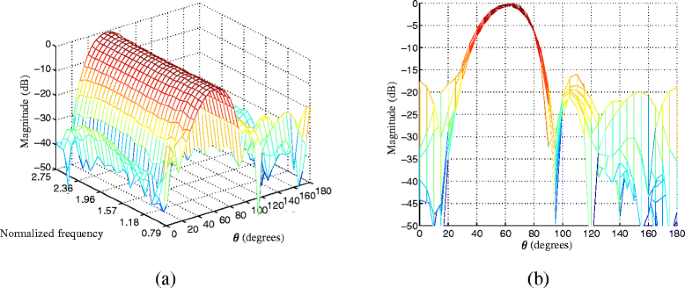Fig. 2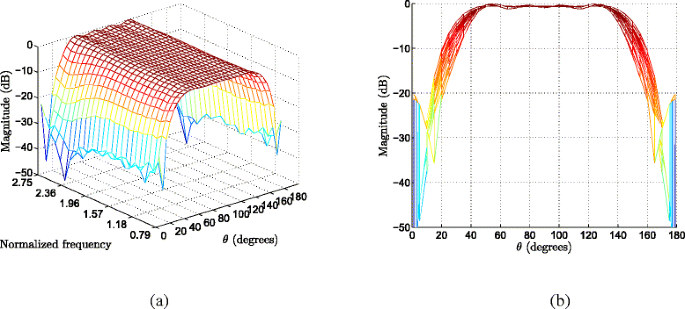Fig. 3

Next, we consider the FSBB design using the WCP optimization in the presence of microphone mismatches. Figure 4 shows the corresponding array response of the FSBB steered to 60°, where the user-defined parameter ε is set to 1.74 according to (11). The simulation result is the average over 100 Monte Carlo trials, i.e., by using 100 random samples of microphone mismatches. As we have discussed above, although the WCP optimization-based criterion has been successfully applied to the robust design of filter-and-sum beamformers, it has failed to work for the design of robust FSBB due to its over-conservativeness. Therefore, the WCP optimization-based criterion may not be suitable for the design of robust FSBB. To justify the overconservativeness of WCP optimization for FSBB design, the array response of the FSBB designed by the less-conservative WCP optimization with the user-defined parameter ε reduced to 0.02 is shown in Fig. 5. Compared with Fig. 4, it can be seen clearly that the beamformer performance can be improved significantly through reducing the effect of conservativeness of WCP optimization.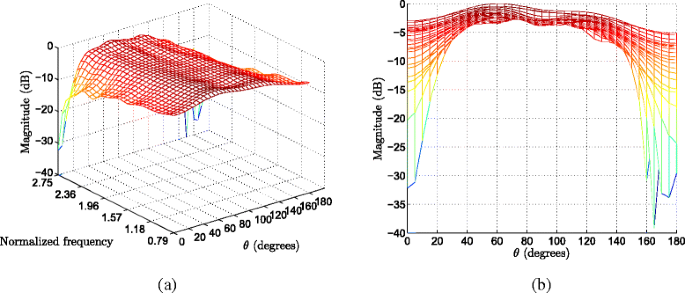Fig. 4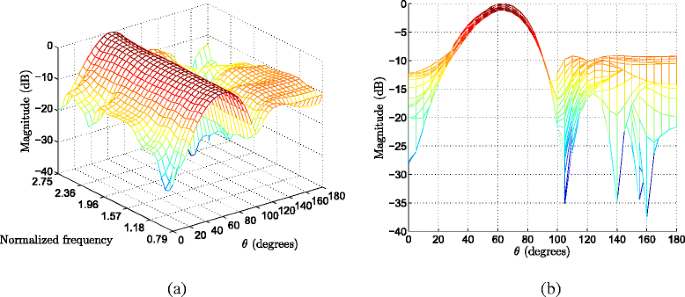Fig. 5

### Example 2: robust design using WCMP optimization with the PRV constraint

In the following, we assume that the mean and variance values of microphone gain and phase errors are all not precisely known due to practical measurement errors. That is, the gain and phase errors are not zero-mean and instead bounded by some small values, i.e., $$|\mathbb {E}\left \{a_{k}\left (\,f,\theta \right)\right \}|\leq 5\times 10^{-6}$$, $$|\mathbb {E}\left \{\gamma _{k}\left (\,f,\theta \right)\right \}|\leq 8.73\times 10^{-6}$$; the variance values are also bounded by Var{a k (f,θ)}≤4.2×10−3 and Var{γ k (f,θ)}≤1.27×10−2, respectively, i.e., each is around five times more than the actual variance of microphone gain/phase errors. All the results are the average over 100 Monte Carlo trials with random samples of microphone mismatches.

Figure 6a and e shows the array response and the PRV of the robust FSBB based on the WCMP optimization with the PRV constraint, respectively, where β=20. While Fig. 6b and f shows the array response and the PRV of the robust FSBB based on the WCMP optimization without the PRV constraint, respectively, i.e. β=0. The steering direction is set to 60°. To see the results more clearly, the associated side views are also presented in Fig. 6c, d, g, and h. Compared with the WCP optimization-based design, i.e., Fig. 4, the design approach using the WCMP optimization performs well in the presence of microphone mismatches. Moreover, by imposing the PRV constraint, the variance of passband array response can be effectively reduced, especially in the low-frequency region. Note also that the PRV of the robust FSBB is nearly invariant with angle θ as demonstrated in Fig. 6g and h, which is consistent with the theoretical finding in Remark 3.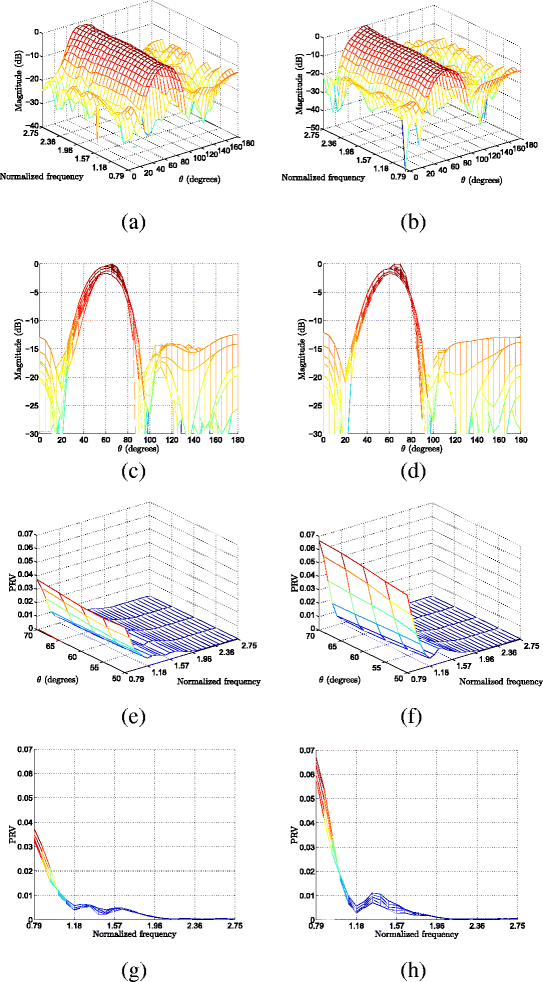Fig. 6

Now, we study the performance of the robust FSBB using WCMP optimization with PRV constraint in the presence of larger microphone mismatch errors. Here, we assume that all microphone gain errors a k (f,θ) have a uniform distribution in [−0.1,0.1], and all microphone phase errors γ k (f,θ) have a uniform distribution in [−π/18,π/18]. Figure 7a and e shows the array response and the PRV of the robust FSBB based on the WCMP optimization with the PRV constraint, respectively, where β=20. While Fig. 7b and f plots the array response and the PRV of the robust FSBB based on the WCMP optimization without the PRV constraint, respectively. The steering direction is set to 60°. For ease of comparison, the associated side views are also presented in Fig. 7c, d, g, and h. From the simulation results, we can see that the robust FSBB still shows satisfactory performance even in the presence of larger microphone mismatch errors. Similar to the above case with smaller microphone mismatches, by imposing the PRV constraint, the variance of passband array response of the FSBB beamformer can also be reduced.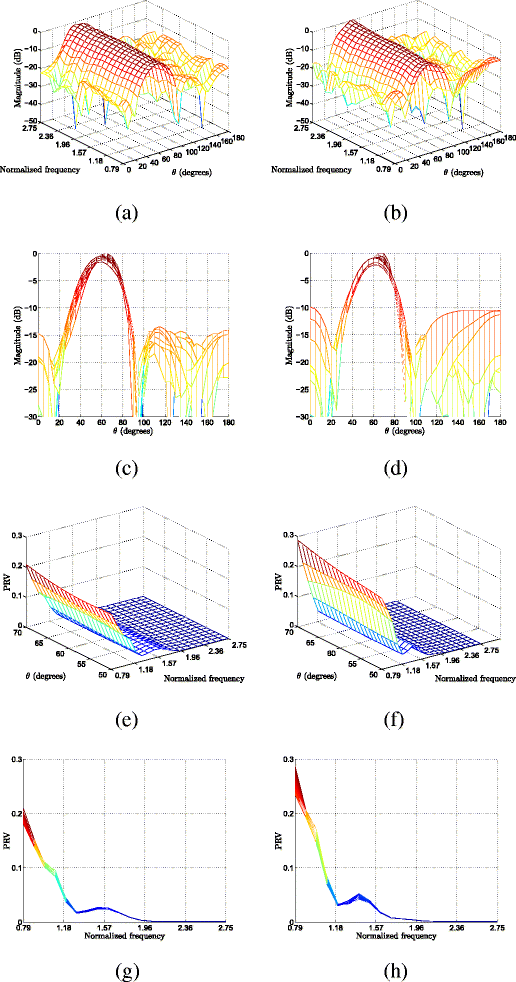Fig. 7

To show the effect of the PRV constraint on the performance of robust FSBB, we first introduce the passband fluctuation , which is defined as the ratio of maximum mean magnitude response to the minimum one in the passband. Passband fluctuation is an indicator of the deviation of the actual mean passband response obtained from the desired flat-top one. Figure 8a, b, and c shows the passband fluctuation, the stopband level, and the average PRV of the robust FSBB with various PRV constraints in the presence of microphone gain errors [−0.05,0.05] and microphone phases errors [−π/36,π/36], where two cases are considered, i.e., ϕ d =60° and 90°. As can be seen from Fig. 8a and c, with more stringent PRV constraint, i.e., increasing the trade-off parameter β, the PRV of the FSBB tends to decrease, while keeping the passband fluctuation at a lower level. However, this is at the cost of sacrificing the stopband level as shown in Fig. 8b. Therefore, a trade-off between the performance of passband and that of the stopband should be considered during design of robust FSBB.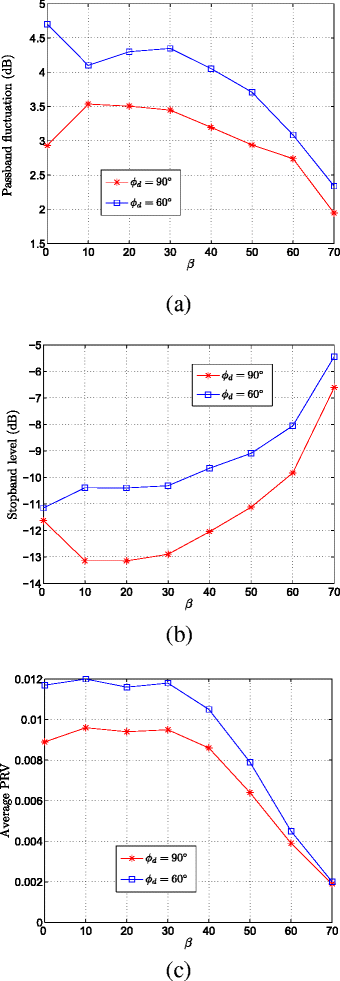Fig. 8

As analyzed above, the PRV of the FSBB is dependent on steering direction. Now, we study the effect of the steering direction on the PRV of the FSBB. Figure 9a and b shows the average PRV of the FSBB versus steering direction ϕ d with β=0 and β=20, respectively. Herein, four FSBBs with different number of microphones K and different FIR tap length N have been considered, i.e., K=7, N=20; K=7, N=30; K=10, N=20; and K=10, N=30. As expected, it can be seen from Fig. 9 that the PRV of the FSBB is varying with steering direction. Interestingly, the average PRV tends to increase with the steering direction deviating from the array broadside.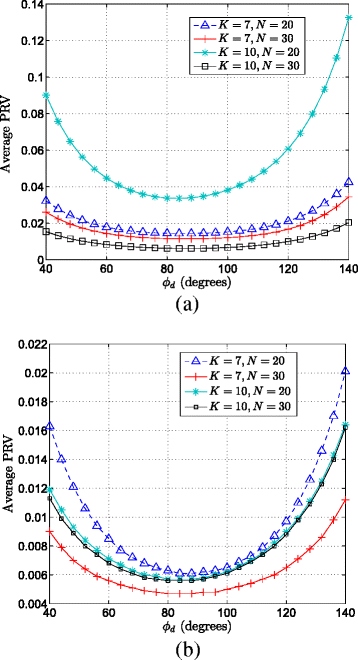Fig. 9

### Example 3: robust design with sparse tap weights

Now, we study the performance of the robust FSBB design with sparse tap weights by using Algorithm III. The user parameters are set as: the trade-off parameter μ=5×10−7, the threshold parameter ξ T =10−6, the parameter for numerical stability ε T =10−6, and the maximum number of iterations L=4. The remaining user parameters are set same as in Example 2. All the results are the average over 100 Monte Carlo trials with random samples of microphone mismatches.

First, we demonstrate the complexity-reducing impact of the sparsity constraint on the robust FSBB. Figure 10 shows the performance comparison of the sparse FSBB and its non-sparse counterpart with N=30, where the steering direction is ϕ d =60°, and there is no PRV constraint (i.e., β=0). Herein, the non-sparse FSBB refers to the FSBB designed by Algorithm II, which has a full active tap weights, i.e., no zero-valued tap weights. For the sparse FSBB, the number of the active weights is reduced to 738, i.e., over 50 % tap weights of the non-sparse FSBB are nullified. The array response of the sparse and non-sparse FSBBs is shown in Fig. 10a and b, while the PRV of the sparse and non-sparse FSBBs is shown in Fig. 10e and f. In order to see the results more clearly, the corresponding side views are also presented in Fig. 10c and d and g and h, respectively. As can be seen from Fig. 10c and d, although over one half of tap weights are nullified, the beampattern of the resultant sparse FSBB has nearly unaffected compared with the beampattern of its non-sparse counterpart. Moreover, the variance of passband array response of the sparse FSBB has only varied slightly compared with that of its non-sparse counterpart, as shown in Fig. 10g and h. Therefore, it has justified our statement that there are redundancy in the tap weights of an FSBB, and a lower-complexity FSBB can be designed via imposing the sparsity constraint without producing a significant degradation of performance.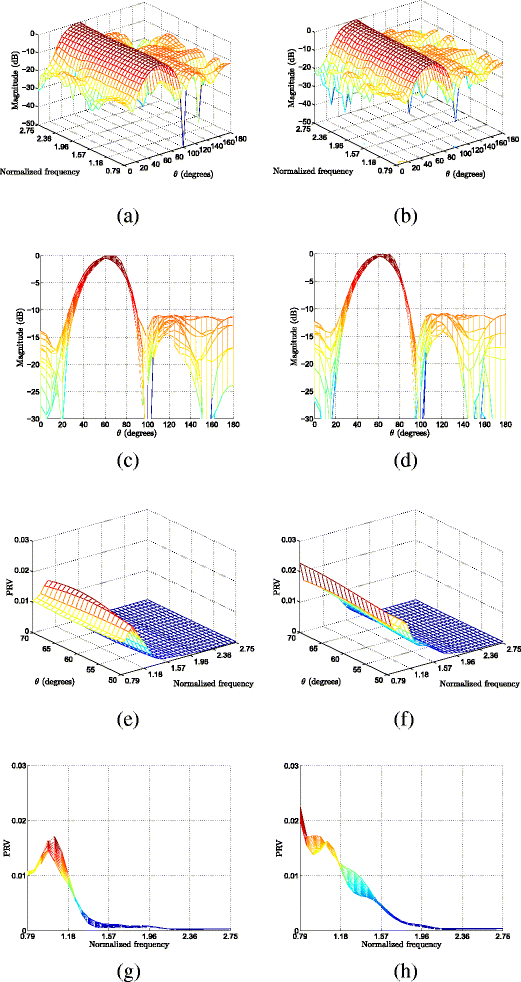Fig. 10

Next, we show another advantage of the sparse FSBB over the non-sparse FSBB with similar computational complexity. Figure 11 shows the performance comparison of sparse and non-sparse FSBBs with a comparable amount of active tap weights, i.e., with a similar computational complexity, where the steering direction is ϕ d =60° and there is no PRV constraint. For the sparse FSBB, the number of the active weights is 738, with N=30. For the non-sparse FSBB, the number of the active weights is 750, with N=15. Note here that, for the purpose of ensuring a fair comparison, the active weights of the sparse FSBB is chosen slightly less than that of the non-sparse FSBB. The array response of the sparse and non-sparse FSBBs is shown in Fig. 11a and b, while the PRV of the sparse and non-sparse FSBBs is shown in Fig. 11e and f. To see the results more clearly, the corresponding side views are also plotted in Fig. 11c and d and g and h, respectively. For the spare FSBB, the stopband level and passband fluctuation are −7.988 and 2.043 dB, respectively, with the average PRV 0.005. For the non-sparse FSBB, the stopband level and passband fluctuation are −7.802 dB and 2.408 dB, respectively, with the average PRV 0.011. Comparatively, the sparse FSBB is superior to the non-sparse FSBB with a similar computational complexity.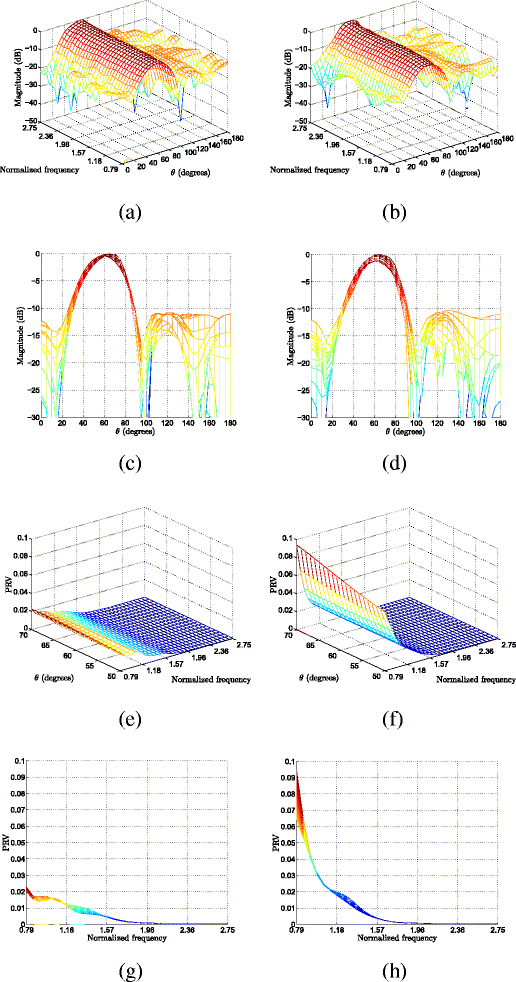Fig. 11

Finally, we consider the effect of the PRV constraint on the robust FSBB with sparse tap weights. Figure 12 shows the performance of the sparse FSBB under various PRV constraints. For comparison, the performance of non-sparse FSBB with a comparable number of active weights is also shown in Fig. 12. Here, the simulation settings are same as in Fig. 11. Moreover, the case with the steering direction ϕ d =90° is also considered. As can be seen from Fig. 12, the sparse FSBB outperforms its non-sparse counterpart under various PRV constraints. Similar to the case of non-sparse FSBB shown in Fig. 8, the PRV of the sparse FSBB will decrease with a more stringent PRV constraint, and this is also at the cost of the stopband level increasing as shown in Fig. 11b.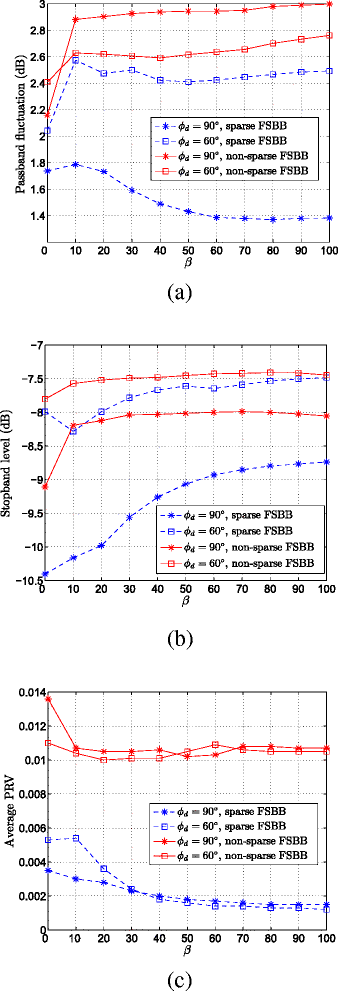Fig. 12

## Conclusions

In this paper, the study of robust FSBB design with sparse tap weights via convex optimization has been conducted by incorporating some priori knowledge of microphone mismatches. It has been shown that due to the over-conservativeness of the WCP optimization criterion, it may become unapplicable to the robust FSBB design, though it has been successfully applied in the robust filter-and-sum beamformer design. When the limited knowledge of mean and variance of microphone mismatches is available, the robust FSBB design approach based on the WCMP optimization with the PRV constraint has been presented. Compared with the WCP optimization-based design, it performs well in the presence of microphone mismatches; moreover, it has the capability of passband stability control of array response. Some insights into the PRV properties of FSBB are also revealed to better understand the robustness characteristic of FSBB. It was also shown in the paper that there exists redundancy in the tap weights of the robust FSBB. To further reduce the computational complexity of the robust FSBB, a two-stage design approach based on the reweighted l 1-norm constraint optimization has been proposed to sparsify the tap weights of robust FSBB. Several design examples have been presented to illustrate the performance of the presented approaches.

## Endnotes

1 The passband is also known as the mainlobe of a beamformer.

2 The stopband is also known as the sidelobe of a beamformer.

## Abbreviations

FSBB:

Farrow-structure-based steerable broadband beamformer

FIR:

finite impulse response filter

WCP:

worst-case performance

WCMP:

worst-case mean performance

WNG:

white noise gain

PRV:

passband response variance

PDF:

probability density function

SOCP:

second-order cone programming

## References

1. 1

M Brandstein, D Ward (eds.), Microphone arrays: signal processing techniques and applications (Springer, Berlin, 2001).

2. 2

BD Van Veen, KM Buckley, Beamforming: a versatile approach to spatial filtering. IEEE ASSP Mag. 5(2), 4–24 (1988).

3. 3

S Doclo, M Moonen, Design of far-field and near-field broadband beamformers using eigenfilters. Signal Process. 83(12), 2641–2673 (2003).

4. 4

S Sriram, P Ashish, J Kees, Beamforming under quantization errors in wireless binaural hearing aids. EURASIP J. Audio Speech Music Process. 2008, 824797 (2008).

5. 5

M Pirro, S Squartini, L Romoli, F Piazza, Stereophonic hands-free communication system based on microphone array fixed beamforming: real-time implementation and evaluation. EURASIP J. Audio Speech Music Process. 2012, 26 (2012).

6. 6

YX Zou, P Wang, YQ Wang, CH Ritz, J Xi, Speech enhancement with an acoustic vector sensor: an effective adaptive beamforming and post-filtering approach. EURASIP J. Audio Speech Music Process. 2014, 17 (2014).

7. 7

RMM Derkx, K Janse, Theoretical analysis of a first-order azimuth-steerable superdirective microphone array. IEEE Trans. Audio Speech Lang. Process. 17(1), 150–162 (2009).

8. 8

CC Lai, S Nordholm, YH Leung, Design of steerable spherical broadband beamformers with flexible sensor configurations. IEEE Trans. Audio Speech Lang. Process. 21(2), 427–438 (2013).

9. 9

CC Lai, S Nordholm, YH Leung, in Proceedings of International Workshop on Acoustic Echo and Noise Control. Design of robust steerable broadband beamformers with spiral arrays and the farrow filter structure (Tel Aviv, 2010).

10. 10

M Kajala, M Hamalainen, Filter-and-sum beamformer with adjustable filter characteristics. Acoustics, Speech, and Signal Processing 2001. Proc. IEEE Int. Conf. Acoust. Speech, Signal Process. 5, 2917–2920 (2001).

11. 11

CC Lai, S Nordholm, YH Leung, in Proceedings IEEE International Conference on Acoustics, Speech, and Signal Processing. Design of robust steerable broadband beamformers incorporating microphone gain and phase error characteristics (Prague, 2011), pp. 101–104.

12. 12

E Mabande, W Kellermann, in Proceedings of International Workshop on Acoustic Echo and Noise Control. Design of robust polynomial beamformers as a convex optimization problem (Tel Aviv, 2010).

13. 13

E Mabande, M Buerger, W Kellermann, in Proceedings IEEE International Conference on Acoustics, Speech, and Signal Processing. Design of robust polynomial beamformers for symmetric arrays (Kyoto, 2012), pp. 1–4.

14. 14

H Wang, H Chen, Y Bao, et al, in Proceedings of IEEE International Conference on Signal Processing, Communication and Computing. Design of steerable and frequency invariant beamformers robust against microphone mismatches (Kunming, 2013), pp. 1–6.

15. 15

S Doclo, M Moonen, Design of broadband beamformers robust against gain and phase errors in the microphone array characteristics. IEEE Trans. Signal Process. 51(10), 2511–2526 (2003).

16. 16

H Chen, W Ser, ZL Yu, Optimal design of nearfield wideband beamformers robust against errors in microphone array characteristics. IEEE Trans. Circuits Syst. I: Regular Papers. 54(9), 1950–1959 (2007).

17. 17

RC Nongpiur, Design of minimax broadband beamformers that are robust to microphone gain, phase, and position errors. IEEE/ACM Trans. Audio Speech Lang. Process. 22(6), 1013–1022 (2014).

18. 18

H Chen, W Ser, J Zhou, Robust nearfield wideband beamformer design using worst case mean performance optimization with passband response variance constraint. Audio Speech Lang. Process. IEEE Trans. 20(5), 1565–1572 (2012).

19. 19

S Boyd, L Vandenberghe, Convex optimization (Cambridge university press, New York, 2009).

20. 20

JF Sturm, Using SeDuMi 1.02, a MATLAB toolbox for optimization over symmetric cones. Optimization Methods Softw. 11(1–4), 625–653 (1999).

21. 21

EJ Candes, MB Wakin, SP Boyd, Enhancing sparsity by reweighted l 1 minimization. J. Fourier Anal. Appl. 14(5–6), 877–905 (2008).

22. 22

M Grant, S Boyd, Y Ye, CVX: Matlab software for disciplined convex programming (2008). http://cvxr.com/cvx/. Accessed 28 May 2015.

Download references

## Acknowledgements

This work was supported by the Fundamental Research Funds for the Central Universities under Grant NS2014041.

## Author information

Authors

### Corresponding author

Correspondence to Huawei Chen.

## Additional information

### Competing interests

The authors declare that they have no competing interests.

## Rights and permissions

Open Access This article is distributed under the terms of the Creative Commons Attribution 4.0 International License (https://creativecommons.org/licenses/by/4.0), which permits use, duplication, adaptation, distribution, and reproduction in any medium or format, as long as you give appropriate credit to the original author(s) and the source, provide a link to the Creative Commons license, and indicate if changes were made.

Reprints and Permissions